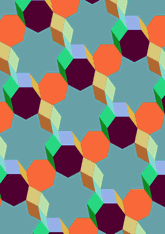# New tilings, Septagonsdata157/R7A

## Geometry

• The symmetry group of the tiling is O (p1).
• All the internal angles of the constituent polygons are a multiple of 25.71 (π/7)°.
• Contains three regular two-pointed star polygons with vertex angle of 25.71 (π/7)°.
• Contains two regular two-pointed star polygons with vertex angle of 51.42 (2π/7)°.
• Contains three regular two-pointed star polygons with vertex angle of 77.14 (3π/7)°.
• Contains two regular heptagons.
• Contains one regular 7-pointed star polygon with vertex angle of 77.14 (3π/7)°.
• The tiling does not satisfy the two-colour condition.
• The tiling is edge-to-edge.
• As drawn, contains about 174 polygons.

## References

Publications referenced:
1. Centred tiling of Not specific. Personal Communication, Information sent to the author, 21c. [pc]

v53